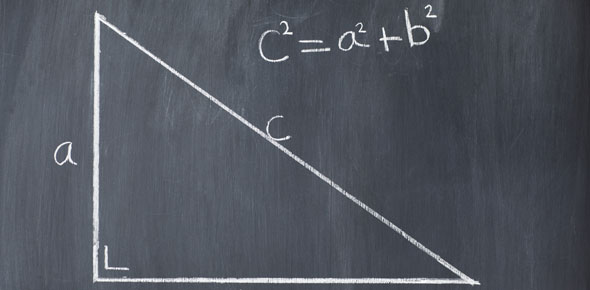# Right Triangle Quiz 4.4.3

7 Questions | Total Attempts: 58SettingsTrigonometry is a branch of mathematics that studies relationships involving lengths and angles of triangles. It comprises of rules, which guide through the study. Take this quiz to find out how much you know. All the best.

• 1.
Find the altitude BD.
• A.

36

• B.

6

• C.

7.5

• D.

15

• E.

12

• 2.
Find the altitude BD.
• A.

81

• B.

24

• C.

30

• D.

9

• E.

Cannot be determined

• 3.
Find the altitude BD to the nearest tenth.
• A.

8.7

• B.

20

• C.

10.5

• D.

6.5

• E.

11.2

• 4.
Find the length of AB to the nearest tenth. Note, you will need to find BD first and then do Pythagorean Theorem.
• A.

5.5

• B.

6.0

• C.

10.8

• D.

9.2

• E.

7.2

• 5.
Find the length of BC. You will need to find BD first and then do Pythagorean Theorem.
• A.

5.5

• B.

6.0

• C.

10.8

• D.

9.2

• E.

7.2

• 6.
Find the length of FG.
• 7.
Using the same triangle from # 7, find GH.
Related TopicsBack to top